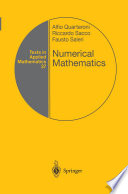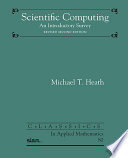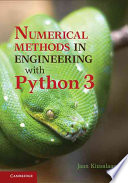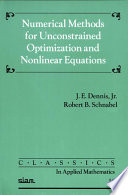### Numerical Mathematics

#### Course Description

Sources of errors in numerical computations. Direct methods for solving systems of linear equations. Polynomial interpolation. Numerical integration and differentiation. Linear least square problems. Root finding for nonlinear equations. Fundamentals of unconstrained optimization.

#### Learning Outcomes

1. distinguish and identify types of errors in numerical computing
2. apply direct methods in solving systems of linear equations
3. identify ill conditioned system of linear equations
4. compute interpolation polynomial and cubic spline for given data and estimate the error of the approximation
5. compute approximation value of a definite integral and estimate error of the approximation
6. describe and apply important methods in solving problems of linear least squares
7. apply derived numerical methods for solving nonlinear equations
8. use fundamental methods of unconstrained optimization
9. use choosen numerical software for solving problems using numerical methods
10. analyze accuracy of obtained results and visualization of numerical solutions

#### Forms of Teaching

Lectures

Lectures are held in two cycles, 3 hours per week

Exercises

Exercises are held in two cycles, 1 hour per week

Partial e-learning

Students will solve online quizzes

Laboratory

During the semester students will work on program tasks

Continuous Assessment Exam
Laboratory Exercises 0 % 15 % 0 % 15 %
Quizzes 0 % 20 % 0 % 0 %
Mid Term Exam: Written 0 % 35 % 0 %
Final Exam: Written 0 % 30 %
Exam: Written 0 % 85 %

#### Week by Week Schedule

1. Sources of Error in Computational Models. Floating point precision and error propagation. Finite differences.
2. The Gaussian Elimination Method (GEM). LU Factorization. Applications. Pivoting Strategies.
3. PLU Factorization of the matrix. Symmetric and Positive Definite Matrices. The Cholesky Factorization.
4. Stability Analysis for Linear Systems. Matrix Norms. The Condition Number of the Matrix. Improving the Accuracy of GEM. Scaling. Iterative refinement.
5. Lagrange Form of the Interpolation Polynomial. The Interpolation Error. Divided Differences. Newton Form of the Interpolation Polynomial.
6. Approximation by splines. Linear and cubic spline. Introduction to numerical integration.
7. Midpoint, Trapezoidal and Simpson rule. Composite Newton-Cotes Formulae. Richardson Extrapolation. Romberg integration. Applications.
8. Midterm exam
9. Linear least squares. Polynomial approximation. Overdetermined linear systems. Normal equations. Sensitivity and conditioning.
10. Matrix Factorization that Solve the Linear Least Square Problem. QR and SVD Decomposition. Applications of the SVD and QR decomposition in solving linear least squares problem
11. Root finding of Nonlinear Equation . The Bisection Method. Fixed-Point Iterations Method.
12. Newton’s Method. Secant method. Numerical solving of nonlinear system. Applications
13. Fundamentals of unconstrained optimization. Direct Search Algorithms. Gradient Methods (the steepest descent). Line Search Techniques. Trust-Region Methods. Conjugate Gradient Method.
14. Quasi-Newton Methods. Large-scale unconstrained optimization. Nonlinear Least-Squares Problems. The Gauss-Newton Method. The Levenberg-Marquardt Method.
15. Final exam

#### Study Programmes

Computing (study)
Free Elective Courses (5. semester)
Electrical Engineering and Information Technology (study)
Free Elective Courses (5. semester)
Audio Technologies and Electroacoustics (profile)
Free Elective Courses (1. semester)
Communication and Space Technologies (profile)
Free Elective Courses (1. semester)
Computational Modelling in Engineering (profile)
Core-elective course (1. semester)
Computer Engineering (profile)
Elective Course of the Profile (1. semester)
Computer Science (profile)
Free Elective Courses (1. semester)
Control Systems and Robotics (profile)
Free Elective Courses (1. semester)
Data Science (profile)
Free Elective Courses (1. semester)
Electrical Power Engineering (profile)
Free Elective Courses (1. semester)
Electric Machines, Drives and Automation (profile)
Free Elective Courses (1. semester)
Electronic and Computer Engineering (profile)
Elective Courses of the Profile (1. semester)
Electronics (profile)
Free Elective Courses (1. semester)
Information and Communication Engineering (profile)
Free Elective Courses (1. semester)
Network Science (profile)
Free Elective Courses (1. semester)
Software Engineering and Information Systems (profile)
Elective Course of the Profile (1. semester)

#### Literature

Milišić, Josipa Pina; Žgaljić Keko, Ana, (2013.), Uvod u numeričku matematiku za inženjere, Element
Z. Drmač, V. Hari, M. Marušić, M. Rogina, S. Singer, S. Singer (2003.), Numerička analiza, PMF-MOAlfio Quarteroni, Riccardo Sacco, Fausto Saleri (2017.), Numerical Mathematics, SpringerMichael T. Heath (2018.), Scientific Computing, SIAMJaan Kiusalaas (2013.), Numerical Methods in Engineering with Python 3, Cambridge University PressJ. E. Dennis, Jr., Robert B. Schnabel (1996.), Numerical Methods for Unconstrained Optimization and Nonlinear Equations, SIAM

#### General

ID 183445
Winter semester
5 ECTS
L1 English Level
L2 e-Learning
45 Lectures
15 Exercises
10 Laboratory exercises